×#### Thank you for registering.

One of our academic counsellors will contact you within 1 working day.

Click to Chat

1800-1023-196

+91-120-4616500

CART 0

• 0

MY CART (5)

Use Coupon: CART20 and get 20% off on all online Study Material

ITEM
DETAILS
MRP
DISCOUNT
FINAL PRICE
Total Price: Rs.

There are no items in this cart.
Continue Shopping• Complete JEE Main/Advanced Course and Test Series
• OFFERED PRICE: Rs. 15,900
• View Details

```Chapter 15: Properties of Triangles Exercise – 15.3

Question: 1

∠CBX is an exterior angle of ∆ABC at B. Name

(ii) the interior opposite angles to exterior ∠CBX

Also, name the interior opposite angles to an exterior angle at A.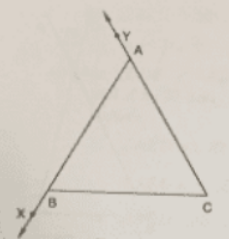Solution:

(i) ∠ABC

(ii) ∠BAC and ∠ACB

Also the interior angles opposite to exterior are ∠ABC and ∠ACB

Question: 2

In the fig, two of the angles are indicated. What are the measures of ∠ACX and ∠ACB?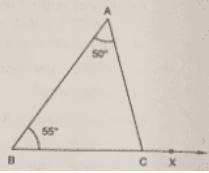Solution:

In ∆ABC, ∠A = 50° and ∠B = 55°

Because of the angle sum property of the triangle, we can say that

∠A + ∠B + ∠C = 180°

50°+ 55°+ ∠C = 180°

Or

∠C = 75°

∠ACB = 75°

∠ACX = 180°− ∠ACB = 180°−75° = 105°

Question: 3

In a triangle, an exterior angle at a vertex is 95° and its one of the interior opposite angles is 55°. Find all the angles of the triangle.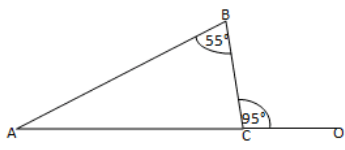Solution:

We know that the sum of interior opposite angles is equal to the exterior angle.

Hence, for the given triangle, we can say that:

∠ABC+ ∠BAC = ∠BCO

55° + ∠BAC = 95°

Or,

∠BAC= 95°– 95°

= ∠BAC = 40°

We also know that the sum of all angles of a triangle is 180°.

Hence, for the given △ABC, we can say that:

∠ABC + ∠BAC + ∠BCA = 180°

55° + 40° + ∠BCA = 180°

Or,

∠BCA = 180° –95°

= ∠BCA = 85°

Question: 4

One of the exterior angles of a triangle is 80°, and the interior opposite angles are equal to each other. What is the measure of each of these two angles?

Solution:

Let us assume that A and B are the two interior opposite angles.

We know that ∠A is equal to ∠B.

We also know that the sum of interior opposite angles is equal to the exterior angle.

Hence, we can say that:

∠A + ∠B = 80°

Or,

∠A +∠A = 80° (∠A= ∠B)

2∠A = 80°

∠A = 40/2 =40°

∠A= ∠B = 40°

Thus, each of the required angles is of 40°.

Question: 5

The exterior angles, obtained on producing the base of a triangle both ways are 104° and 136°. Find all the angles of the triangle.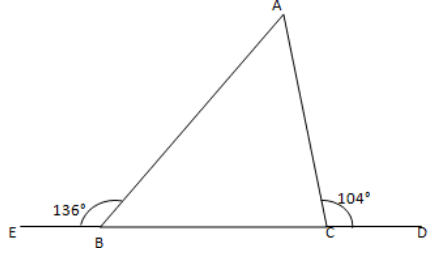Solution:

In the given figure, ∠ABE and ∠ABC form a linear pair.

∠ABE + ∠ABC =180°

∠ABC = 180°– 136°

∠ABC = 44°

We can also see that ∠ACD and ∠ACB form a linear pair.

∠ACD + ∠ACB = 180°

∠AUB = 180°– 104°

∠ACB = 76°

We know that the sum of interior opposite angles is equal to the exterior angle.

Therefore, we can say that:

∠BAC + ∠ABC = 104°

∠BAC = 104°– 44° = 60°

Thus,

∠ACE = 76° and ∠BAC = 60°

Question: 6

In Fig, the sides BC, CA and BA of a ∆ABC have been produced to D, E and F respectively. If ∠ACD = 105° and ∠EAF = 45°; find all the angles of the ∆ABCSolution:

In a ∆ABC, ∠BAC and ∠EAF are vertically opposite angles.

Hence, we can say that:

∠BAC = ∠EAF = 45°

Considering the exterior angle property, we can say that:

∠BAC + ∠ABC = ∠ACD = 105°

∠ABC = 105°– 45° = 60°

Because of the angle sum property of the triangle, we can say that:

∠ABC + ∠ACS +∠BAC = 180°

∠ACB = 75°

Therefore, the angles are 45°, 65° and 75°.

Question: 7

In Figure, AC perpendicular to CE and C ∠A: ∠B: ∠C= 3: 2: 1. Find the value of ∠ECD.Solution:

In the given triangle, the angles are in the ratio 3: 2: 1.

Let the angles of the triangle be 3x, 2x and x.

Because of the angle sum property of the triangle, we can say that:

3x + 2x + x = 180°

6x = 180°

Or,

x = 30° … (i)

Also, ∠ACB + ∠ACE + ∠ECD = 180°

x + 90° + ∠ECD = 180° (∠ACE = 90°)

∠ECD = 60° [From (i)]

Question: 8

A student when asked to measure two exterior angles of ∆ABC observed that the exterior angles at A and B are of 103° and 74° respectively. Is this possible? Why or why not?

Solution:

Here,

Internal angle at A + External angle at A = 180°

Internal angle at A + 103° =180°

Internal angle at A = 77°

Internal angle at B + External angle at B = 180°

Internal angle at B + 74° = 180°

Internal angle at B = 106°

Sum of internal angles at A and B = 77° + 106° =183°

It means that the sum of internal angles at A and B is greater than 180°, which cannot be possible.

Question: 9

In Figure, AD and CF are respectively perpendiculars to sides BC and AB of ∆ABC. If ∠FCD = 50°, find ∠BAD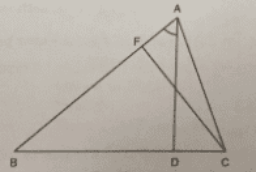Solution:

We know that the sum of all angles of a triangle is 180°

Therefore, for the given ∆FCB, we can say that:

∠FCB + ∠CBF + ∠BFC = 180°

50° + ∠CBF + 90°= 180°

Or,

∠CBF = 180° – 50°– 90° = 40° … (i)

Using the above rule for ∆ABD, we can say that:

∠ABD + ∠BDA + ∠BAD = 180°

∠BAD = 180° – 90°– 40° = 50° [from (i)]

Question: 10

In Figure, measures of some angles are indicated. Find the value of x.Solution:

Here,

∠AED + 120° = 180° (Linear pair)

∠AED = 180°– 120° = 60°

We know that the sum of all angles of a triangle is 180°.

Therefore, for △ADE, we can say that:

∠ADE + ∠AED + ∠DAE = 180°

Or,

∠ADE = 180°– 60°– 30° = 90°

From the given figure, we can also say that:

∠FDC + 90° = 180° (Linear pair)

∠FDC = 180°– 90° = 90°

Using the above rule for △CDF, we can say that:

∠CDF + ∠DCF + ∠DFC = 180°

90° + ∠DCF + 60° =180°

∠DCF = 180°−60°− 90°= 30°

Also,

∠DCF + x = 180° (Linear pair)

30° + x = 180°

Or,

x = 180°– 30° = 150°

Question: 11

In Figure, ABC is a right triangle right angled at A. D lies on BA produced and DE perpendicular to BC intersecting AC at F. If ∠AFE = 130°, find

(i) ∠BDE

(ii) ∠BCA

(iii) ∠ABC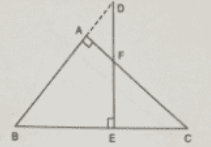Solution:

(i) Here,

∠BAF + ∠FAD = 180° (Linear pair)

∠FAD = 180°- ∠BAF = 180°– 90° = 90°

Also,

∠ADF = 130°− 90° = 40°

(ii) We know that the sum of all the angles of a triangle is 180°.

Therefore, for ∆BDE, we can say that:

∠BDE + ∠BED + ∠DBE = 180°.

∠DBE = 180°– ∠BDE ∠BED = 180°− 90°− 40°= 50° — (i)

Also,

∠FAD = ∠ABC + ∠ACB (Exterior angle property)

90° = 50° + ∠ACB

Or,

∠ACB = 90°– 50° = 40°

(iii) ∠ABC = ∠DBE = 50° [From (i)]

Question: 12

ABC is a triangle in which ∠B = ∠C and ray AX bisects the exterior angle DAC. If ∠DAX = 70°. Find ∠ACB.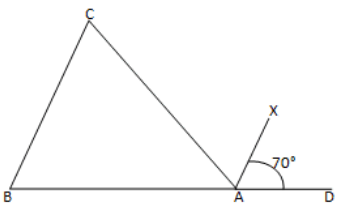Solution:

Here,

∠CAX = ∠DAX (AX bisects ∠CAD)

∠CAX =70°

∠CAX +∠DAX + ∠CAB =180°

70°+ 70° + ∠CAB =180°

∠CAB =180° –140°

∠CAB =40°

∠ACB + ∠CBA + ∠CAB = 180° (Sum of the angles of ∆ABC)

∠ACB + ∠ACB+ 40° = 180° (∠C = ∠B)

2∠ACB = 180°– 40°

∠ACB = 140/2

∠ACB = 70°

Question: 13

The side BC of ∆ABC is produced to a point D. The bisector of ∠A meets side BC in L. If ∠ABC= 30° and ∠ACD = 115°, find ∠ALC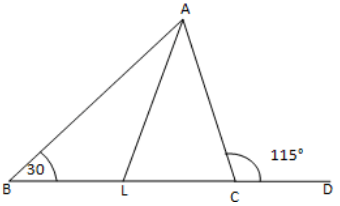Solution:

∠ACD and ∠ACL make a linear pair.

∠ACD+ ∠ACB = 180°

115° + ∠ACB =180°

∠ACB = 180°– 115°

∠ACB = 65°

We know that the sum of all angles of a triangle is 180°.

Therefore, for ∆ABC, we can say that:

∠ABC + ∠BAC + ∠ACB = 180°

30° + ∠BAC + 65° = 180°

Or,

∠BAC = 85°

∠LAC = ∠BAC/2 = 85/2

Using the above rule for ∆ALC, we can say that:

∠ALC + ∠LAC + ∠ACL = 180°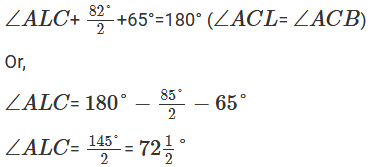Question: 14

D is a point on the side BC of ∆ABC. A line PDQ through D, meets side AC in P and AB produced at Q. If ∠A = 80°, ∠ABC = 60° and ∠PDC = 15°, find

(i) ∠AQD

(ii) ∠APDSolution:

∠ABD and ∠QBD form a linear pair.

∠ABC + ∠QBC =180°

60° + ∠QBC = 180°

∠QBC = 120°

∠PDC = ∠BDQ (Vertically opposite angles)

∠BDQ = 75°

In ∆QBD:

∠QBD + ∠QDB + ∠BDQ = 180° (Sum of angles of ∆QBD)

120°+ 15° + ∠BQD = 180°

∠BQD = 180°– 135°

∠BQD = 45°

∠AQD = ∠BQD = 45°

In ∆AQP:

∠QAP + ∠AQP + ∠APQ = 180° (Sum of angles of ∆AQP)

80° + 45° + ∠APQ = 180°

∠APQ = 55°

∠APD = ∠APQ

Question: 15

Explain the concept of interior and exterior angles and in each of the figures given below. Find x and ySolution:

The interior angles of a triangle are the three angle elements inside the triangle.

The exterior angles are formed by extending the sides of a triangle, and if the side of a triangle is produced, the exterior angle so formed is equal to the sum of the two interior opposite angles.

Using these definitions, we will obtain the values of x and y.

(I) From the given figure, we can see that:

∠ACB + x = 180° (Linear pair)

75°+ x = 180°

Or,

x = 105°

We know that the sum of all angles of a triangle is 180°.

Therefore, for ∆ABC, we can say that:

∠BAC+ ∠ABC +∠ACB = 180°

40°+ y +75° = 180°

Or,

y = 65°

(ii) x + 80°= 180° (Linear pair)

= x = 100°

In ∆ABC:

x+ y+ 30° = 180° (Angle sum property)

100° + 30° + y = 180°

= y = 50°

(iii) We know that the sum of all angles of a triangle is 180°.

Therefore, for ∆ACD, we can say that:

30° + 100° + y = 180°

Or,

y = 50°

∠ACB + 100° = 180°

∠ACB = 80° … (i)

Using the above rule for ∆ACD, we can say that:

x + 45° + 80° = 180°

= x = 55°

(iv) We know that the sum of all angles of a triangle is 180°.

Therefore, for ∆DBC, we can say that:

30° + 50° + ∠DBC = 180°

∠DBC = 100°

x + ∠DBC = 180° (Linear pair)

x = 80°

And,

y = 30° + 80° = 110° (Exterior angle property)

Question: 16

Compute the value of x in each of the following figuresSolution:

(i) From the given figure, we can say that:

∠ACD + ∠ACB = 180° (Linear pair)

Or,

∠ACB = 180°– 112° = 68°

We can also say that:

∠BAE + ∠BAC = 180° (Linear pair)

Or,

∠BAC = 180°– 120° = 60°

We know that the sum of all angles of a triangle is 180°.

Therefore, for ∆ABC:

x + ∠BAC + ∠ACB = 180°

x = 180°– 60°– 68° = 52°

= x = 52°

(ii) From the given figure, we can say that:

∠ABC + 120° = 180° (Linear pair)

∠ABC = 60°

We can also say that:

∠ACB+ 110° = 180° (Linear pair)

∠ACB = 70°

We know that the sum of all angles of a triangle is 180°.

Therefore, for ∆ABC:

x + ∠ABC + ∠ACB = 180°

x = 50°

(iii) From the given figure, we can see that:

We know that the sum of all the angles of a triangle is 180°.

Therefore, for ∆DEC:

x + 40°+ 52° = 180°

= x = 88°

(iv) In the given figure, we have a quadrilateral whose sum of all angles is 360°.

Thus,

35° + 45° + 50° + reflex ∠ADC = 360°

Or,

230° + x = 360° (A complete angle)

= x = 130°
```### Course Features

• 728 Video Lectures
• Revision Notes
• Previous Year Papers
• Mind Map
• Study Planner
• NCERT Solutions
• Discussion Forum
• Test paper with Video Solution